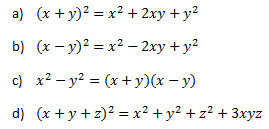# Test Paper for Polynomial Class 9 Maths

Here we are trying to give the polynomials class 9 test papers with answers. It tests the basic concepts and at the same makes the student comfortable with the questions

Question 1
Which of these is a polynomial
(a) $\sqrt {y} + 1$
(b) $\sqrt {2} + x +x^2$
(c) $y+ \frac {1}{y} + y^2$
(d) $\frac {5 \sqrt x + 6x^3/2}{\sqrt x}$
(e) $\frac {x+1}{x-1}$
(f) $x \sqrt {2}$

Question 2
True and false
(a) The degree of non-zero constant polynomail is zero
(b) The degree of the zero polynomial is well defined
(c) A binomial can have atmost two terms
(d) A binomial may have degree 6
(e) A polynomial cannot have more than one zero
(f) The degree of the sum of two polynomials each of degree 6 is always 6.
(g) 0 and 2 are the zeroes of y2- 2y

## Multiple choice Questions

Question 3
If $p + q+ r = 0$, then $p^3 + q^3 + r^3$ is equal to
(a)0
(b)3pqr
(c)2pqr
(d)pqr

Question 4
Which of these identities is not true?Question 5
Find the coefficent of x2 in (x -1)(9x2 - 3x + 1)
(a)12
(b)-3
(c) 9
(d) -12

Question 6
If $x + \frac {1}{x}=3$, then the value $x^2 + \frac {1}{x^2}$ is
(a) 7
(b) 4
(c) 1
(d) 3

Question 7
If x61 + 61 is divided by x + 1, the remainder is
(a) 0
(b) 1
(c) 60
(d) 59

Question 8
Find the value of a, if x - a is a factor of x3- ax2 + 2x + a - 1
(a) 2/3
(b) 1/3
(c) 1
(d) 3

Question 9
if $x + \frac {1}{x}=2$ , then the value of $x^{10}+ \frac {1}{x^{10}}$ is
(a) 2
(b) 0
(c) 1
(d) -1

Question 10
Degree of the quotient polynomials cannot be
(a) 0
(b) greater then degree of the reminder
(c) greater than degree of dividend
(d) None of the above

## Factorization questions

Question 11
Factorize the below expression
(a) 49x2 + 70xy + 25y2
(b) 4y2+ 20y + 25

Question 12
(c) 16x2+ 4y2 + 9z2- 16xy - 12yz + 24xz
(d) 2x3- 3x2- 17x + 30

## Summary

This Class 9 Maths Test Paper for Polynomials is prepared keeping in mind the latest syllabus of CBSE . This has been designed in a way to improve the academic performance of the students. If you find mistakes , please do provide the feedback on the mail. You can download this test as pdf also as below